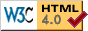# Meteors and TWA Flight 800

This was an unpublished letter to the editor of the New York Times, September 28, 1996.

A series of letters recently have been debating the probability that TWA Flight 800 was downed by a meteor (Sept. 19, 24, and 28). What these letters seem to have ignored is the fact that we already know that the flight “fell out of the sky.” The probability that the flight was downed by a meteor would be the probability of a plane that “fell out of the sky” being hit by a meteor, not the probability of any flight being hit by a meteor.

Suppose that the probability of a flight falling out of the sky is between 1 in 10 million and 1 in 100 million and the probability of a flight being hit by a meteor is 1 in 10 billion. (These figures are my own rough estimates based partially on statistics from the Sept. 19 letter.) Then the probability that a meteor downed the flight, once it is already known it was downed, would be between 1 in 100 and 1 in 1000, not 1 in 10 billion.

An interesting extreme case that may make this more understandable is the following: Suppose that all flights that fall from the sky were hit by meteors. If that were true, then the probability that a downed flight was hit by a meteor would be 1 in 1, not 1 in 10 billion.

L. David Baron(Back to Views, David Baron)